Java 的 Comparator 对多个排序条件的处理？

年薪达不到25W大数据工程师、拿不到Offer全额退款->>>public Class Employee {

public int level;  //级别
public int salary; //工资
public int years;  //入职年数

}

Comparator<Employee> cp_by_default = new Comparator<Employee>(){
@Override
public int compare(Employee a1, Employee a2) {
int a = a2.level - a1.level;
if(a > 0)
return a;
a = a2.salary - a1.salary;
if(a > 0)
return a;
return a2.years - a1.years;
}
};

1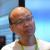package net.oschina.tester;

import java.util.ArrayList;
import java.util.Collections;
import java.util.Comparator;
import java.util.List;

/**
* 多个排序条件测试
* @author Winter Lau (http://my.oschina.net/javayou)
* @date 2011-6-2 上午9:56:27
*/
public class Employee {

public static void main(String[] args) {
List<Employee> objs = new ArrayList<Employee>(){{
}};
Collections.sort(objs, comparator);
System.out.println("No\tLevel\tSalary\tYears\n=============================");
for(Employee a : objs)
System.out.printf("%d\t%d\t%d\t%d\n",a.id,a.level,a.salary,a.year);
}

public Employee(int id, int level, int salary, int year){
this.id = id;
this.level = level;
this.salary = salary;
this.year = year;
}

public int id;
public int level;
public int salary;
public int year;

private final static Comparator<Employee> comparator = new Comparator<Employee>(){
@Override
public int compare(Employee a1, Employee a2) {
int cr = 0;
int a = a2.level - a1.level;
if(a != 0)
cr = (a>0)?3:-1;
else{
a = a2.salary - a1.salary;
if(a != 0)
cr = (a>0)?2:-2;
else{
a = (int)(a2.year - a1.year);
if(a != 0)
cr = (a>0)?1:-3;
}
}
//System.out.printf("compare(%d,%d)=%d\n", a1.getId(), a2.getId(), cr);
return cr;
}
};

}
1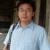int x = a1.level - a2.level
int y = a1.salary - a2.salary
int z = a1.years - a2.years
if(x==0){
if(y==0){
return z;
}
return y;
}
return x;

1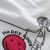package com.lewole;

import java.util.Comparator;
import java.util.List;
import java.util.ArrayList;

/**
* Sort <code>List</code> by multiple conditions.<br>
* It's like SQL 'Order by' clause. Input multiple <code>Comparator</code>
* @author jimmy ideajava@gmail.com
* @param <T>
*/
public class GroupComparator<T> implements Comparator<T>{
private List<Comparator<T>> comparators = new ArrayList<Comparator<T>>();
public GroupComparator(Comparator<T> first, Comparator<T> second){
}

public void addComparator(Comparator<T> comparator){
}

public int compare(T t1, T t2){
for (Comparator<T> comparator : comparators)
{
int returnValue = comparator.compare(t1, t2);

if (returnValue != 0)
return returnValue;
}

return 0;
}
}

Comparator<TopicBean> sort1 = new Comparator<TopicBean>(){
public int compare(TopicBean t1, TopicBean t2) {
return t1.getDown() - t2.getDown();
}
};

Comparator<TopicBean> sort2 = new Comparator<TopicBean>(){
public int compare(TopicBean t1, TopicBean t2) {
return t1.getCatId() - t2.getCatId();
}
};

Comparator<TopicBean> sort3 = new Comparator<TopicBean>(){
public int compare(TopicBean t1, TopicBean t2) {
return t1.getUp()- t2.getUp();
}
};
GroupComparator<TopicBean> gc = new GroupComparator<TopicBean>(sort1,sort2);
..............

Collections.sort(topics,gc);

0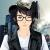import java.util.ArrayList;
import java.util.Collections;
import java.util.Comparator;
import java.util.List;

public class Test
{
public void sortT(List<Employee> list)
{
Collections.sort(list, new Comparator<Employee>()
{
@Override
public int compare(Employee a1, Employee a2)
{
int a = a2.level - a1.level;
if (a > 0)
return a;
a = a2.salary - a1.salary;
if (a > 0)
return a;
return a2.years - a1.years;
}
});
}

public void sortA(List<Employee> list)
{
Collections.sort(list, new Comparator<Employee>()
{
@Override
public int compare(Employee a1, Employee a2)
{
int x = a1.level - a2.level;
int y = a1.salary - a2.salary;
int z = a1.years - a2.years;
if (x == 0)
{
if (y == 0)
{
return -z;
}
return -y;
}
return -x;
}
});
}

public static void main(String[] args)
{
List<Employee> list = new ArrayList<Employee>();
list.add(new Employee(1, 1, 2, 3));
list.add(new Employee(2, 1, 1, 3));
list.add(new Employee(3, 1, 2, 2));
list.add(new Employee(4, 2, 2, 3));
list.add(new Employee(5, 2, 1, 3));
list.add(new Employee(6, 2, 2, 1));
Test tt = new Test();
tt.sortT(list);
for (Employee e : list)
{
System.out.println(e);
}
System.out.println("===================");
tt.sortT(list);
for (Employee e : list)
{
System.out.println(e);
}
}
}

class Employee
{
int id;
int level;
int salary;
int years;

public Employee(int id, int level, int salary, int years)
{
this.id = id;
this.level = level;
this.salary = salary;
this.years = years;
}

public String toString()
{
return id + "  " + level + "   " + salary + "   " + years;
}
}
00package fx.sunjoy;

import java.util.ArrayList;
import java.util.Collections;
import java.util.Comparator;
import java.util.List;

public class Employee {

public static void main(String[] args) {
List<Employee> objs = new ArrayList<Employee>(){{
}};
Collections.sort(objs, comparator);
System.out.println("No\tLevel\tSalary\tYears\n=============================");
for(Employee a : objs)
System.out.printf("%d\t%d\t%d\t%d\n",a.id,a.level,a.salary,a.year);
System.out.println("");
Collections.sort(objs, sjycmp);
System.out.println("No\tLevel\tSalary\tYears\n=============================");
for(Employee a : objs)
System.out.printf("%d\t%d\t%d\t%d\n",a.id,a.level,a.salary,a.year);

}

public Employee(int id, int level, int salary, int year){
this.id = id;
this.level = level;
this.salary = salary;
this.year = year;
}

public int id;
public int level;
public int salary;
public int year;

private final static Comparator<Employee> sjycmp = new Comparator<Employee>() {

@Override
public int compare(Employee a1, Employee a2) {
int x = a1.level - a2.level;
int y = a1.salary - a2.salary;
int z = a1.year - a2.year;
if(x==0){
if(y==0){
return -z;
}
return -y;
}
return -x;
}

};

private final static Comparator<Employee> comparator = new Comparator<Employee>(){
@Override
public int compare(Employee a1, Employee a2) {
int cr = 0;
int a = a2.level - a1.level;
if(a != 0)
cr = (a>0)?3:-1;
else{
a = a2.salary - a1.salary;
if(a != 0)
cr = (a>0)?2:-2;
else{
a = (int)(a2.year - a1.year);
if(a != 0)
cr = (a>0)?1:-3;
}
}
//System.out.printf("compare(%d,%d)=%d\n", a1.getId(), a2.getId(), cr);
return cr;
}
};

}0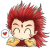private static void mergeSort(Object[] src,
Object[] dest,
int low, int high, int off,
Comparator c) {
int length = high - low;

// Insertion sort on smallest arrays
if (length < INSERTIONSORT_THRESHOLD) {
for (int i=low; i<high; i++)
for (int j=i; j>low && c.compare(dest[j-1], dest[j])>0; j--)
swap(dest, j, j-1);
return;
}

// Recursively sort halves of dest into src
int destLow  = low;
int destHigh = high;
low  += off;
high += off;
int mid = (low + high) >>> 1;
mergeSort(dest, src, low, mid, -off, c);
mergeSort(dest, src, mid, high, -off, c);

// If list is already sorted, just copy from src to dest.  This is an
// optimization that results in faster sorts for nearly ordered lists.
if (c.compare(src[mid-1], src[mid]) <= 0) {
System.arraycopy(src, low, dest, destLow, length);
return;
}

// Merge sorted halves (now in src) into dest
for(int i = destLow, p = low, q = mid; i < destHigh; i++) {
if (q >= high || p < mid && c.compare(src[p], src[q]) <= 0)
dest[i] = src[p++];
else
dest[i] = src[q++];
}
}

0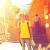import java.util.ArrayList;
import java.util.Collections;
import java.util.Comparator;

/**
* User：ThinerZQ
* Email：thinerzq@gmail.com
* Date：2016/9/23 14:37
* Project：Test
* Package：PACKAGE_NAME
*/
public class TestCompartor {
public static void main(String[] args) {

ArrayList<Person> persons = new ArrayList<Person>();
for (int i = 0; i <persons.size(); i++) {
System.out.println(persons.get(i));
}
System.out.println();
Collections.sort(persons, new Comparator<Person>() {
//级别，年份，薪资排序降序，
public int compare(Person o1, Person o2) {
if (o1.level == o2.level){
if (o1.salary == o2.salary){
return o2.years - o1.years;
}else{
return o2.salary - o1.salary;
}
}else{
return o2.level - o1.level;
}
}
});
for (int i = 0; i <persons.size(); i++) {
System.out.println(persons.get(i));
}
}
static class Person{
public int level;  //级别
public int salary; //工资
public int years;  //入职年数

@Override
public String toString() {
return "Person{" +
"level=" + level +
", salary=" + salary +
", years=" + years +
'}';
}
public Person(int level, int salary, int years) {
this.level = level;
this.salary = salary;
this.years = years;
}
}
}

0

引用来自“601097836”的评论

import java.util.ArrayList;
import java.util.Collections;
import java.util.Comparator;

/**
* User：ThinerZQ
* Email：thinerzq@gmail.com
* Date：2016/9/23 14:37
* Project：Test
* Package：PACKAGE_NAME
*/
public class TestCompartor {
public static void main(String[] args) {

ArrayList<Person> persons = new ArrayList<Person>();
for (int i = 0; i <persons.size(); i++) {
System.out.println(persons.get(i));
}
System.out.println();
Collections.sort(persons, new Comparator<Person>() {
//级别，年份，薪资排序降序，
public int compare(Person o1, Person o2) {
if (o1.level == o2.level){
if (o1.salary == o2.salary){
return o2.years - o1.years;
}else{
return o2.salary - o1.salary;
}
}else{
return o2.level - o1.level;
}
}
});
for (int i = 0; i <persons.size(); i++) {
System.out.println(persons.get(i));
}
}
static class Person{
public int level;  //级别
public int salary; //工资
public int years;  //入职年数

@Override
public String toString() {
return "Person{" +
"level=" + level +
", salary=" + salary +
", years=" + years +
'}';
}
public Person(int level, int salary, int years) {
this.level = level;
this.salary = salary;
this.years = years;
}
}
}